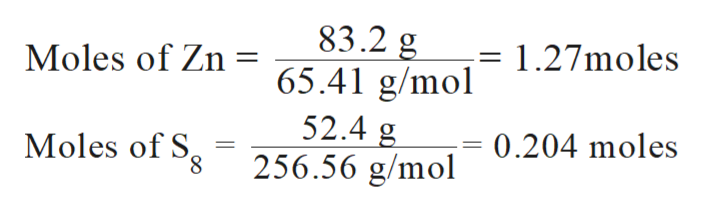# When 83.2g of Zn reacts with 52.4g of S(sub8), 104.4g of ZnS forms. What is the percent yield of ZnS using the following unbalanced equation? Zn(s)+ Ssub8 (s) = ZnS

Question
14 views

When 83.2g of Zn reacts with 52.4g of S(sub8), 104.4g of ZnS forms. What is the percent yield of ZnS using the following unbalanced equation?

Zn(s)+ Ssub8 (s) = ZnS

check_circle

Step 1

In chemistry, there are many ways to check the deviation of experimental data from the accurate value. These different methods provide the difference between accuracy and precisions of the experimental data which can be further used to calculate the error percentage in the experiment.

Step 2

The balance equation for the formation of ZnS from Zn and S8:

Step 3

Mass of Zn  = 83.2 g

Mass of S8 = 52.4 g

Molar mass of Zn  = 65.41 g/mol

Molar mass of...help_outlineImage Transcriptionclose83.2 g Moles of Zn 65.41 g/mol 1.27moles 52.4 g Moles of S 256.56 g/mol 0.204 moles fullscreen

### Want to see the full answer?

See Solution

#### Want to see this answer and more?

Solutions are written by subject experts who are available 24/7. Questions are typically answered within 1 hour.*

See Solution
*Response times may vary by subject and question.
Tagged in

### Chemistry